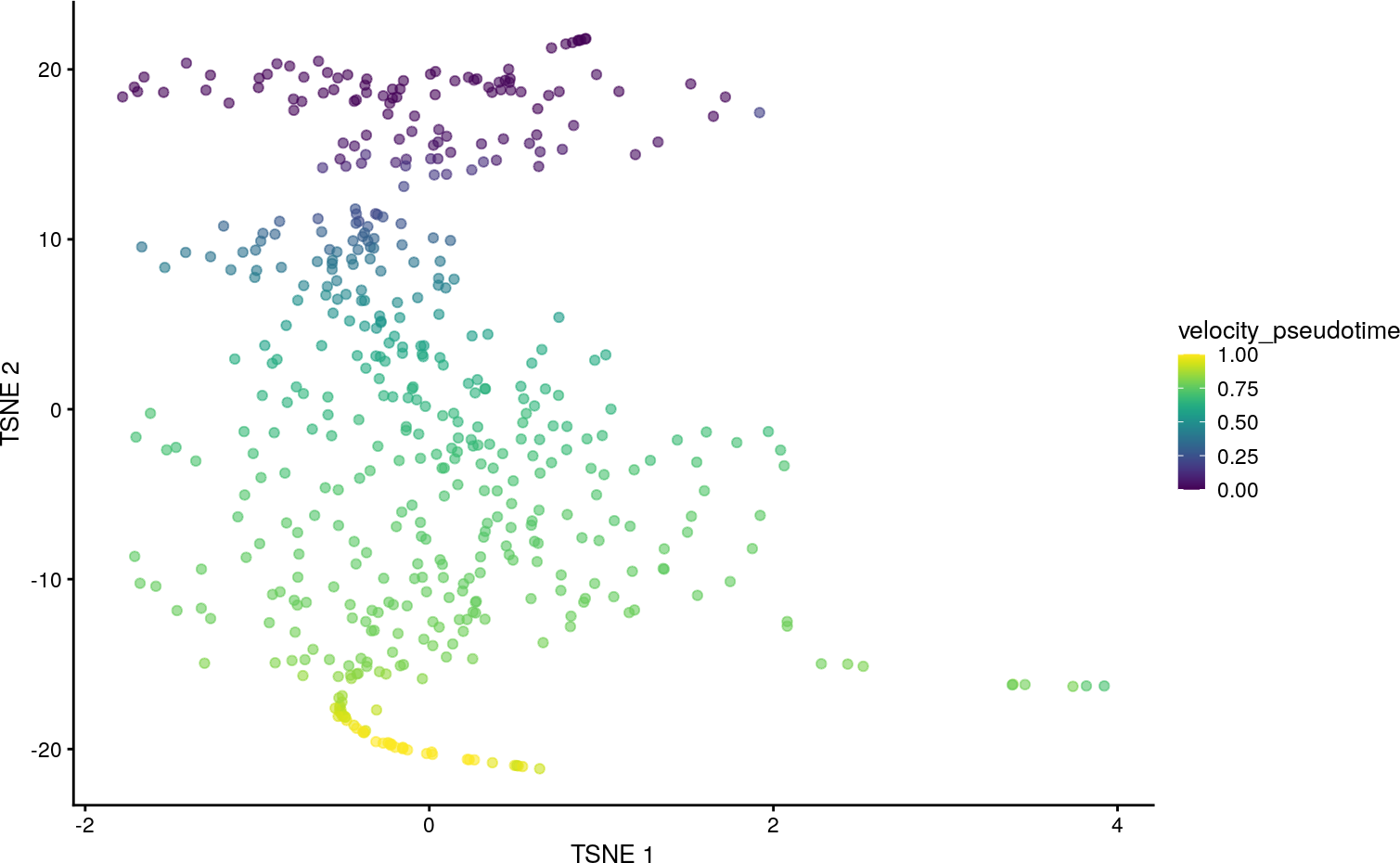# 1 Overview

This package provides a lightweight interface between the Bioconductor `SingleCellExperiment` data structure and the scvelo Python package for RNA velocity calculations. The interface is comparable to that of many other `SingleCellExperiment`-compatible functions, allowing users to plug in RNA velocity calculations into the existing Bioconductor analysis framework. To demonstrate, we will use a data set from Hermann et al. (2018), provided via the scRNAseq package. This data set contains gene-wise estimates of spliced and unspliced UMI counts for 2,325 mouse spermatogenic cells.

``````library(scRNAseq)
sce <- HermannSpermatogenesisData()
sce``````
``````## class: SingleCellExperiment
## dim: 54448 2325
## metadata(0):
## assays(2): spliced unspliced
## rownames(54448): ENSMUSG00000102693.1 ENSMUSG00000064842.1 ...
##   ENSMUSG00000064369.1 ENSMUSG00000064372.1
## rowData names(0):
## colnames(2325): CCCATACTCCGAAGAG AATCCAGTCATCTGCC ... ATCCACCCACCACCAG
##   ATTGGTGGTTACCGAT
## colData names(1): celltype
## reducedDimNames(0):
## altExpNames(0):``````

# 2 Downsampling for demonstration

The full data set requires up to 12 GB of memory for the example usage presented in this vignette. For demonstration purposes, we downsample the data set to the first 500 cells. Feel free to skip this downsampling step if you have access to sufficient memory.

``sce <- sce[, 1:500]``

# 3 Basic workflow

We assume that feature selection has already been performed by the user using any method (see here for some suggestions). We will use the log-expression method from the scran method here.

``````library(scuttle)
sce <- logNormCounts(sce, assay.type=1)

library(scran)
dec <- modelGeneVar(sce)
top.hvgs <- getTopHVGs(dec, n=2000)``````

We can plug these choices into the `scvelo()` function with our `SingleCellExperiment` object. Here, we will use the `"spliced"` count matrix as a proxy for the typical exonic count matrix. While this count matrix is not required for the velocity estimation in itself, it is used internally to retrieve the principal component space that is used by scvelo to find nearest neighbors. There are some subtle differences between the spliced count matrix and the typical exonic count matrix - see `?scvelo` for some commentary about this - but the spliced counts are generally a satisfactory replacement. By default, `scvelo()` uses the steady-state approach to estimate velocities. The stochastic and dynamical models implemented in scvelo are accessible as well, by modifying the `mode` argument.

``````library(velociraptor)
velo.out <- scvelo(sce, subset.row=top.hvgs, assay.X="spliced")
velo.out``````
``````## class: SingleCellExperiment
## dim: 2000 500
## metadata(4): neighbors velocity_params velocity_graph
##   velocity_graph_neg
## assays(6): X spliced ... Mu velocity
## rownames(2000): ENSMUSG00000117819.1 ENSMUSG00000081984.3 ...
##   ENSMUSG00000022965.8 ENSMUSG00000094660.2
## rowData names(3): velocity_gamma velocity_r2 velocity_genes
## colnames(500): CCCATACTCCGAAGAG AATCCAGTCATCTGCC ... CACCTTGTCGTAGGAG
##   TTCCCAGAGACTAAGT
## colData names(7): velocity_self_transition root_cells ...
##   velocity_confidence velocity_confidence_transition
## reducedDimNames(1): X_pca
## altExpNames(0):``````

The `scvelo()` function produces a `SingleCellExperiment` containing all of the outputs of the calculation in Python. Of particular interest is the `velocity_pseudotime` vector that captures the relative progression of each cell along the biological process driving the velocity vectors. We can visualize this effect below in a \(t\)-SNE plot generated by scater on the top HVGs.

``````library(scater)

set.seed(100)
sce <- runPCA(sce, subset_row=top.hvgs)
sce <- runTSNE(sce, dimred="PCA")

sce\$velocity_pseudotime <- velo.out\$velocity_pseudotime
plotTSNE(sce, colour_by="velocity_pseudotime")``````It is also straightforward to embed the velocity vectors into our desired low-dimensional space, as shown below for the \(t\)-SNE coordinates. This uses a grid-based approach to summarize the per-cell vectors into local representatives for effective visualization.

``````embedded <- embedVelocity(reducedDim(sce, "TSNE"), velo.out)
grid.df <- gridVectors(reducedDim(sce, "TSNE"), embedded)

library(ggplot2)
plotTSNE(sce, colour_by="velocity_pseudotime") +
geom_segment(data=grid.df, mapping=aes(x=start.1, y=start.2,
xend=end.1, yend=end.2), arrow=arrow(length=unit(0.05, "inches")))``````